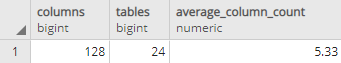## Find number of columns in PostgreSQL database

Article for:

Query returns basic statistics of numbers of columns in a database.

## Query

``````select count(*) as columns,
count(distinct t.table_schema || '.' || t.table_name) as tables,
round ((count(*)::decimal
/ count(distinct t.table_schema || '.' || t.table_name)), 2)
as average_column_count
from information_schema.tables t
left join information_schema.columns c on c.table_schema = t.table_schema
and c.table_name = t.table_name
where t.table_schema not in ('information_schema', 'pg_catalog')
and table_type = 'BASE TABLE';
``````

## Columns

• columns - total number of columns in a database
• tables - number of tables in a database
• average_column_count - average number of columns in a table in a database

## Rows

• Query returns just one row

## Sample results